# What is energy Energy and Energy Resources Energy

• Slides: 36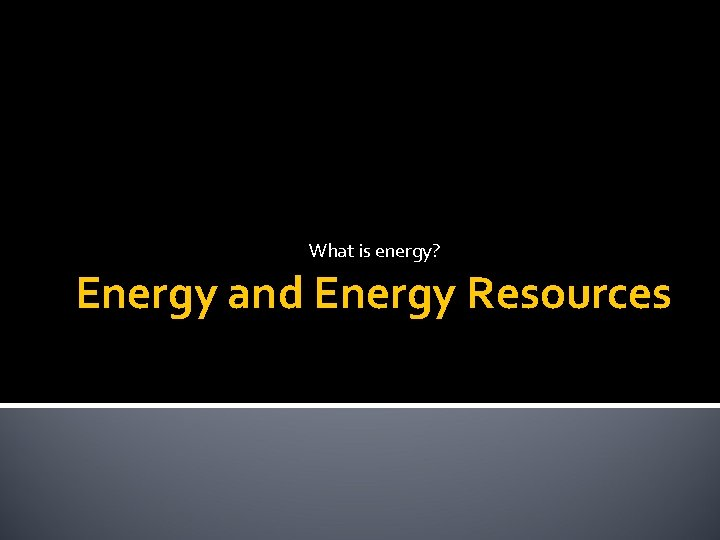What is energy? Energy and Energy Resources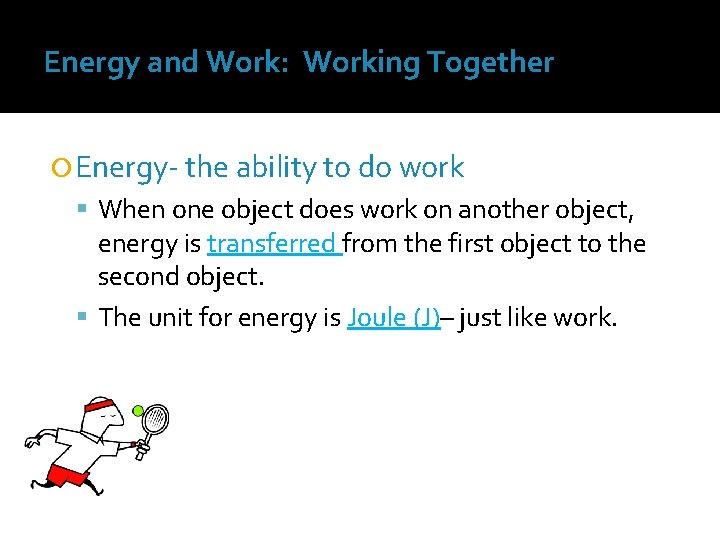Energy and Work: Working Together Energy- the ability to do work When one object does work on another object, energy is transferred from the first object to the second object. The unit for energy is Joule (J)– just like work.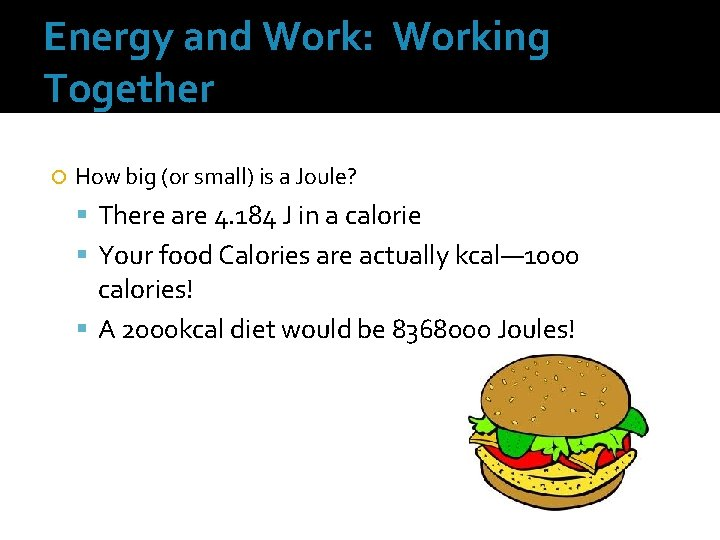Energy and Work: Working Together How big (or small) is a Joule? There are 4. 184 J in a calorie Your food Calories are actually kcal— 1000 calories! A 2000 kcal diet would be 8368000 Joules!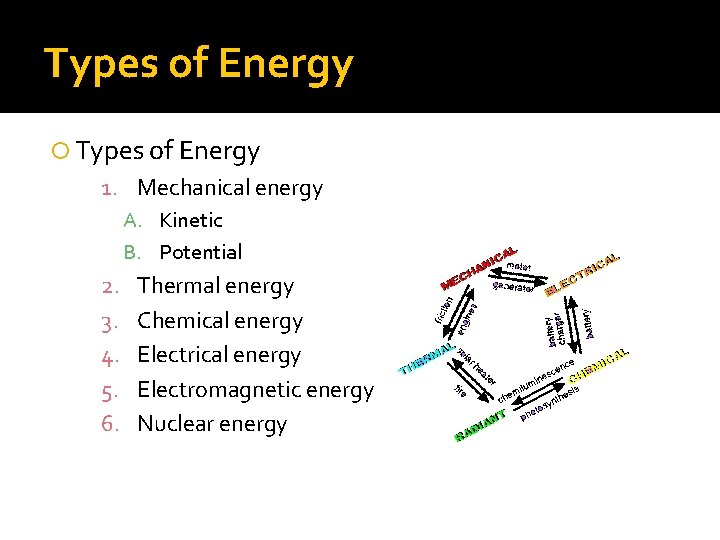Types of Energy 1. Mechanical energy A. Kinetic B. Potential 2. 3. 4. 5. 6. Thermal energy Chemical energy Electromagnetic energy Nuclear energy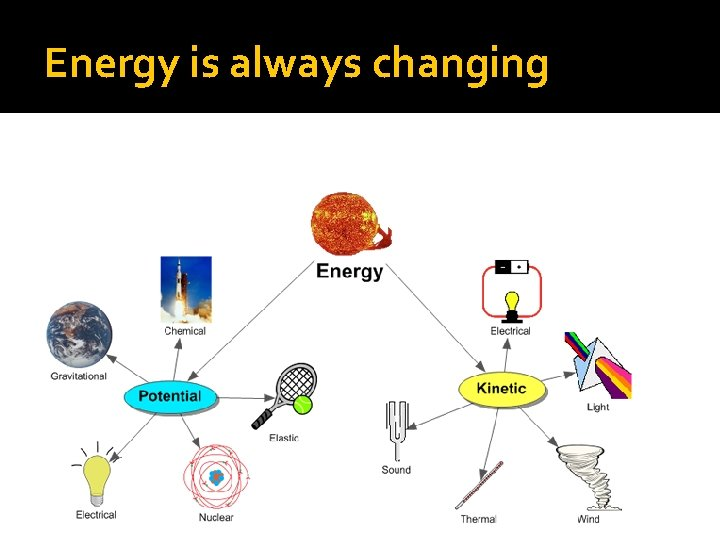Energy is always changing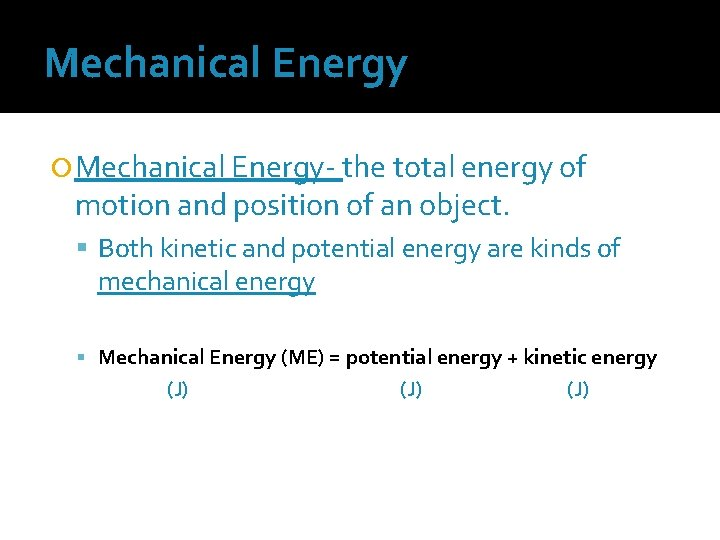Mechanical Energy Mechanical Energy- the total energy of motion and position of an object. Both kinetic and potential energy are kinds of mechanical energy Mechanical Energy (ME) = potential energy + kinetic energy (J) (J)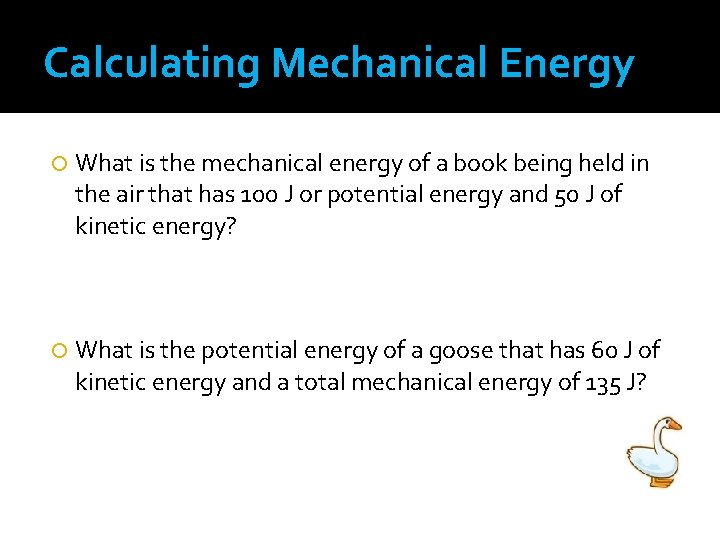Calculating Mechanical Energy What is the mechanical energy of a book being held in the air that has 100 J or potential energy and 50 J of kinetic energy? What is the potential energy of a goose that has 60 J of kinetic energy and a total mechanical energy of 135 J?Kinetic Energy Kinetic Energy- energy in motion All moving objects have kinetic energy Kinetic Energy = KE = mv 2 2 ▪ m = mass (Kg) v = m/s ▪ Velocity has a greater effect on kinetic energy than mass does (because it’s squared)Kinetic Energy Calculations What is the kinetic energy of a car that has a mass of 1, 200 Kg and is moving at a speed of 20 m/s? What is the kinetic energy of a car that has a mass of 2, 400 kg and is moving at 20 m/s? How does this kinetic energy of the car in the above example compare?Kinetic Energy Calculations A 50 kg cheetah has a KE of 18, 000 J. How fast is the cheetah running?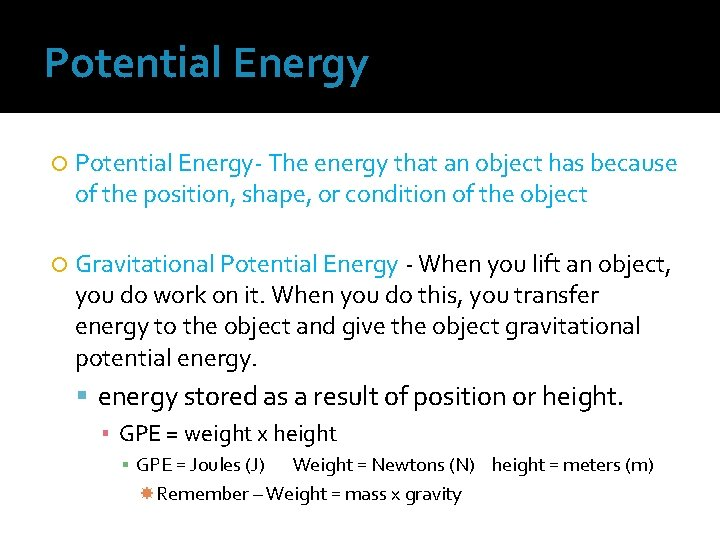Potential Energy Potential Energy- The energy that an object has because of the position, shape, or condition of the object Gravitational Potential Energy - When you lift an object, you do work on it. When you do this, you transfer energy to the object and give the object gravitational potential energy stored as a result of position or height. ▪ GPE = weight x height ▪ GPE = Joules (J) Weight = Newtons (N) height = meters (m) Remember – Weight = mass x gravity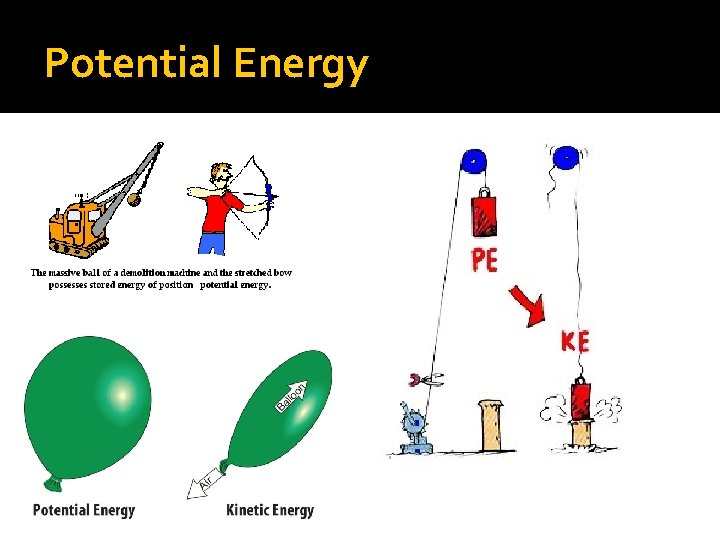Potential EnergyPotential Energy Calculations What is the gravitational potential energy of a book with a weight of 13 N at a height of 1. 5 m off the ground? What is the gravitational potential energy of a cat that weighs 40 N standing on a table that is 0. 8 m above the ground?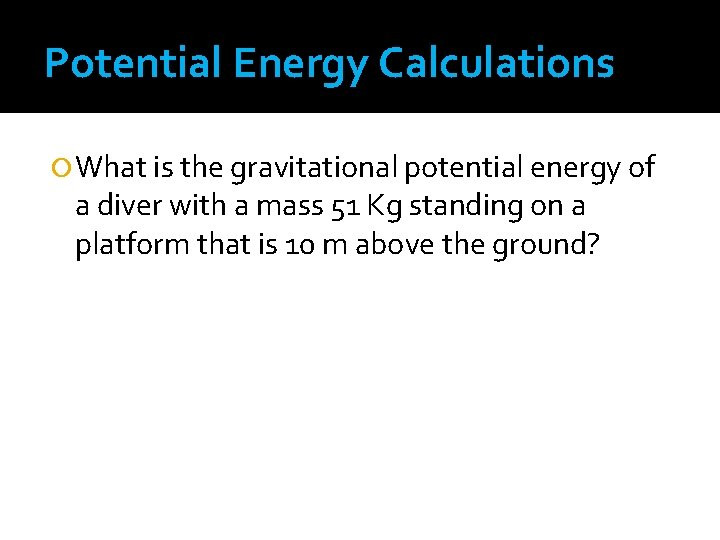Potential Energy Calculations What is the gravitational potential energy of a diver with a mass 51 Kg standing on a platform that is 10 m above the ground?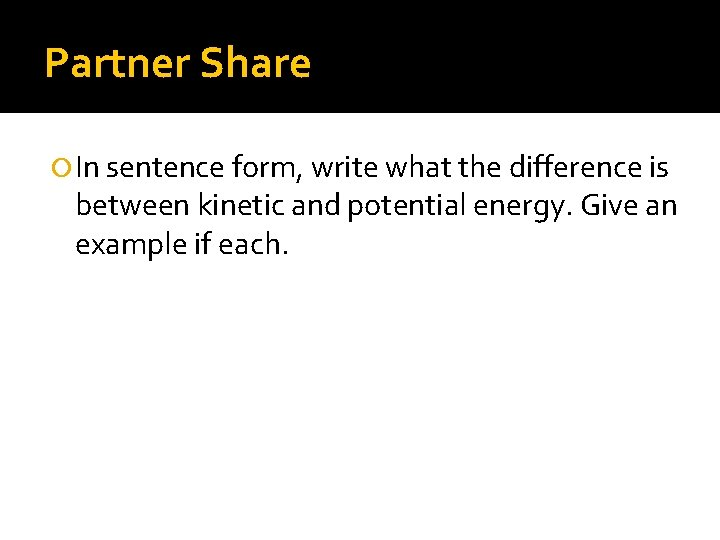Partner Share In sentence form, write what the difference is between kinetic and potential energy. Give an example if each.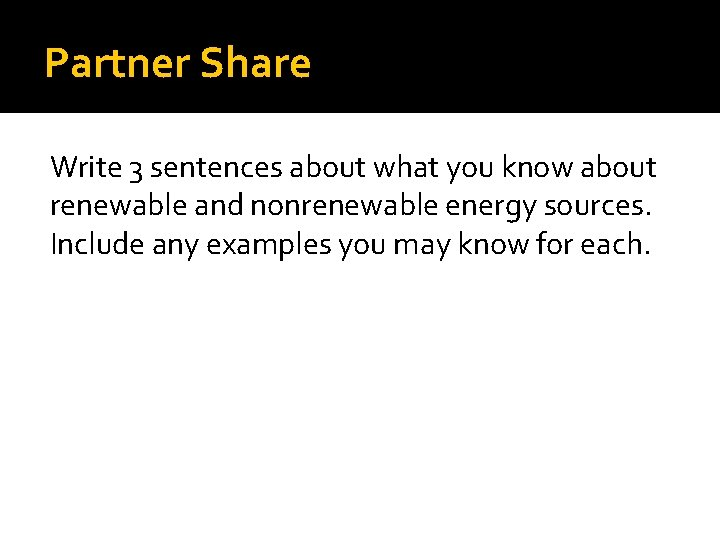Partner Share Write 3 sentences about what you know about renewable and nonrenewable energy sources. Include any examples you may know for each.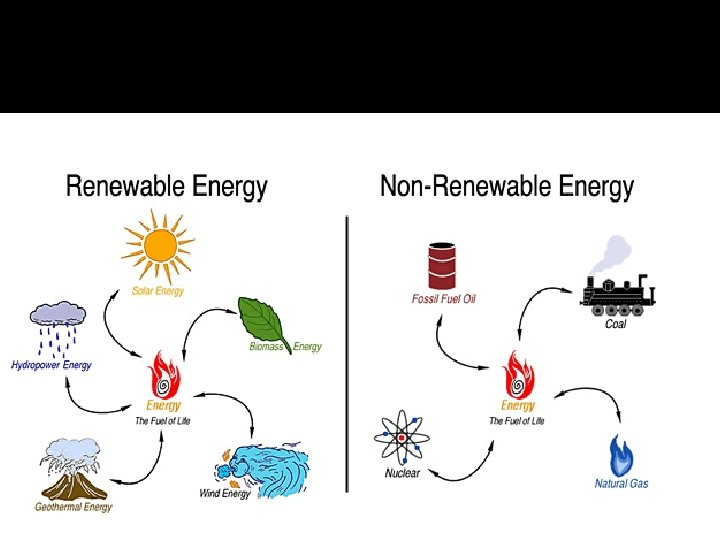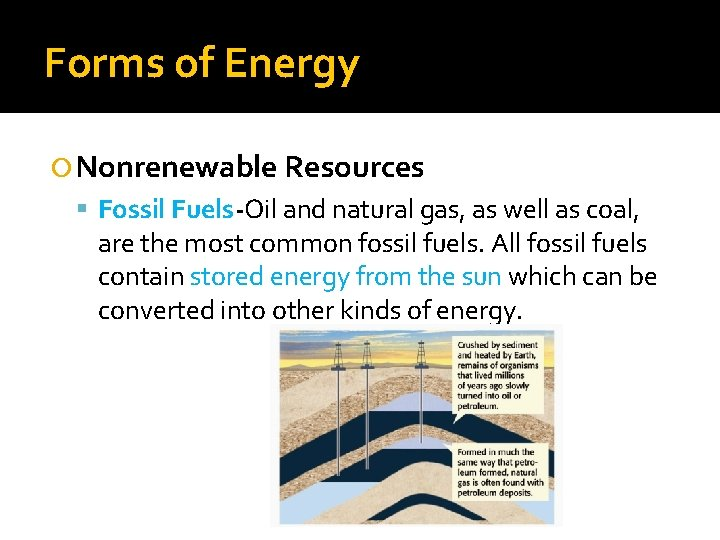Forms of Energy Nonrenewable Resources Fossil Fuels-Oil and natural gas, as well as coal, are the most common fossil fuels. All fossil fuels contain stored energy from the sun which can be converted into other kinds of energy.Fossil Fuels Burning coal is a common way to generate electrical energy. • Petroleum is used to make gasoline, wax, and plastics. • Natural gas is often used in home heating. • • The United States’ primary source of electrical energy is generated by burning fossil fuels.Creating the energy we use How do we make electricity from these resources? Electric generators convert the chemical energy in fossil fuels into electrical energy by the process shown below. An electric generator converts energy of one type into another type of energy.Forms of Energy Nonrenewable Resources cont’d _______The energy that comes from the nucleus of an atom. Nuclear fission is a process of releasing energy through splitting nuclei apart. Nuclear Fusion releases energy when a less massive nuclei combine to form a more massive nucleus.Forms of Energy Renewable Resources Solar -Sunlight can be changed into electrical energy through solar cells. Hydroelectric -The potential energy of water in a reservoir can be changed into kinetic energy as the water flows through a dam.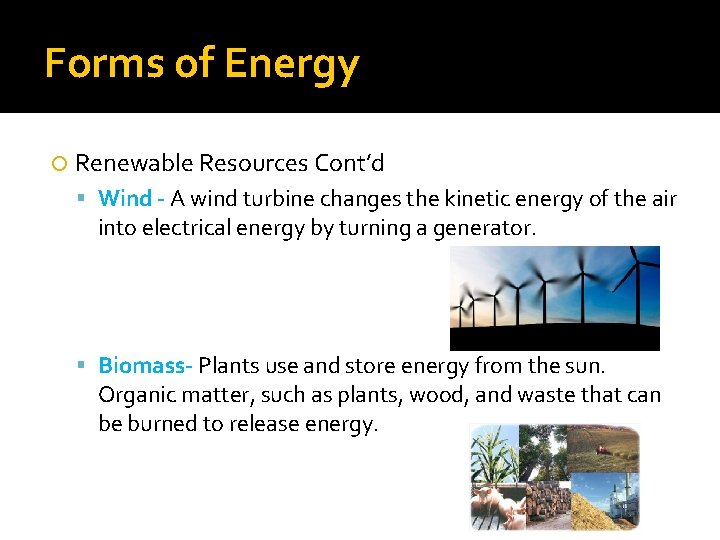Forms of Energy Renewable Resources Cont’d Wind - A wind turbine changes the kinetic energy of the air into electrical energy by turning a generator. Biomass- Plants use and store energy from the sun. Organic matter, such as plants, wood, and waste that can be burned to release energy.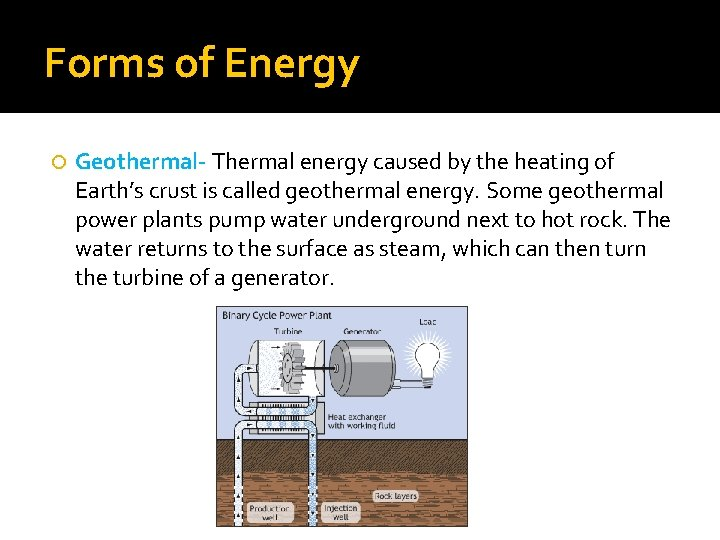Forms of Energy Geothermal- Thermal energy caused by the heating of Earth’s crust is called geothermal energy. Some geothermal power plants pump water underground next to hot rock. The water returns to the surface as steam, which can then turn the turbine of a generator.Other Forms of Energy Thermal energy is all of the kinetic energy due to random motion of the particles that make up an object.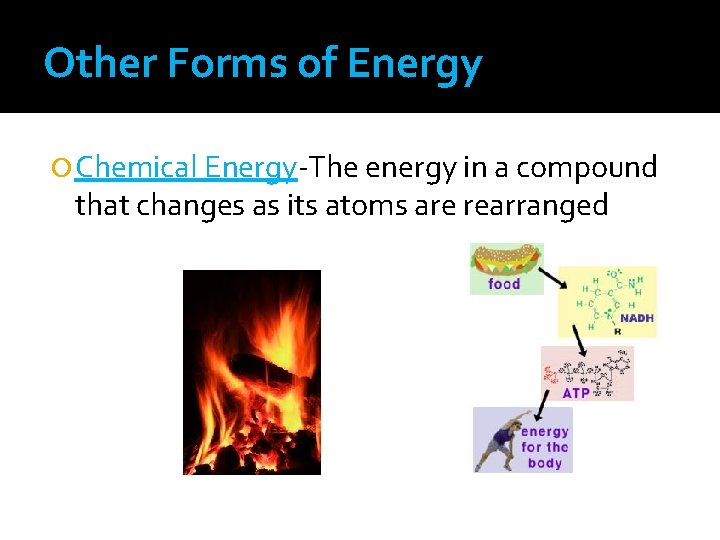Other Forms of Energy Chemical Energy-The energy in a compound that changes as its atoms are rearranged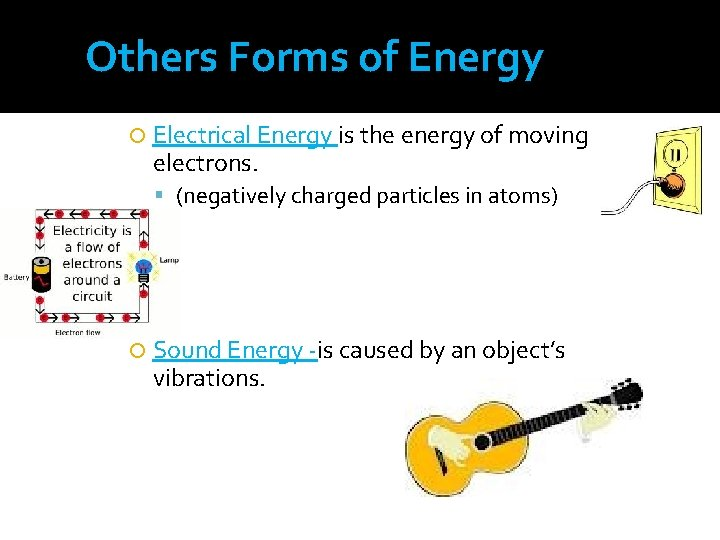Others Forms of Energy Electrical Energy is the energy of moving electrons. (negatively charged particles in atoms) Sound Energy -is caused by an object’s vibrations.Others Forms of Energy Light Energy Produced by the vibrations of electrically charged particles.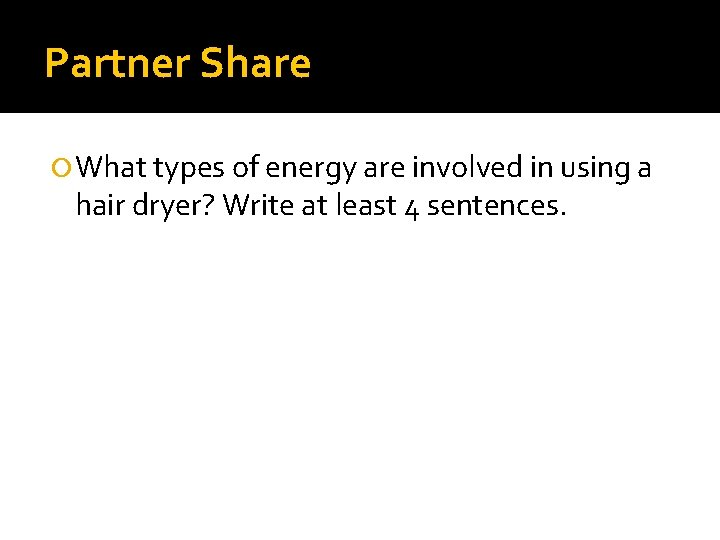Partner Share What types of energy are involved in using a hair dryer? Write at least 4 sentences.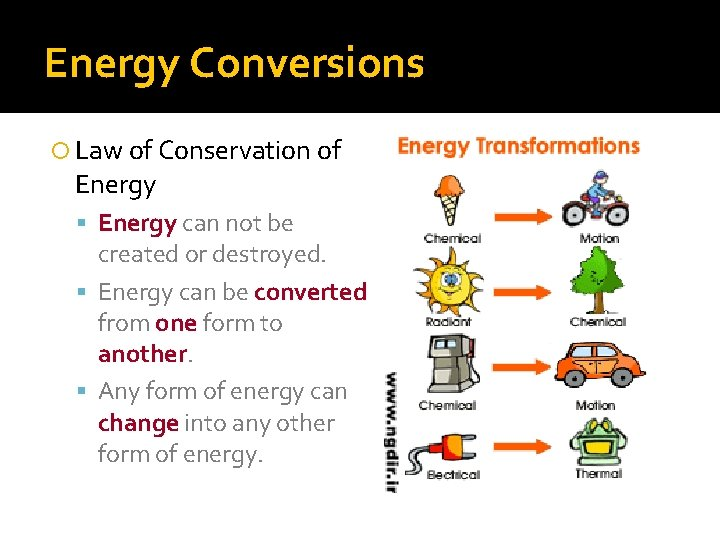Energy Conversions Law of Conservation of Energy can not be created or destroyed. Energy can be converted from one form to another. Any form of energy can change into any other form of energy.In energy conversions, some energy is always lost as heat (Thermal energy). After energy conversions, you always end up with the same total amount of energy as the original amount of potential energy.Where Does Energy go? 3: Conservation of Energy Friction-A force that opposes motion between two surfaces that are touching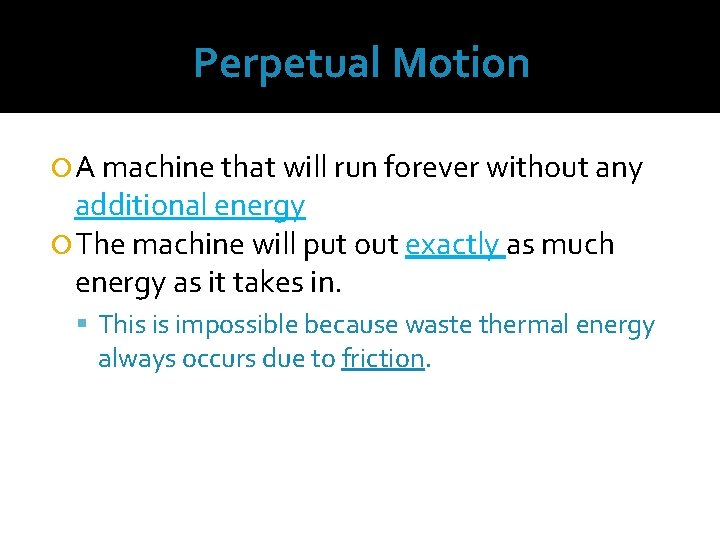Perpetual Motion A machine that will run forever without any additional energy The machine will put out exactly as much energy as it takes in. This is impossible because waste thermal energy always occurs due to friction.Making Conversions Efficient Energy Efficiency - is a comparison of the amount of energy before a conversion with the amount of useful energy after a conversion More efficient = less friction/less lost energyPartner Share Why is there always energy “lost” when there is a transfer of energy? Where does the energy go?